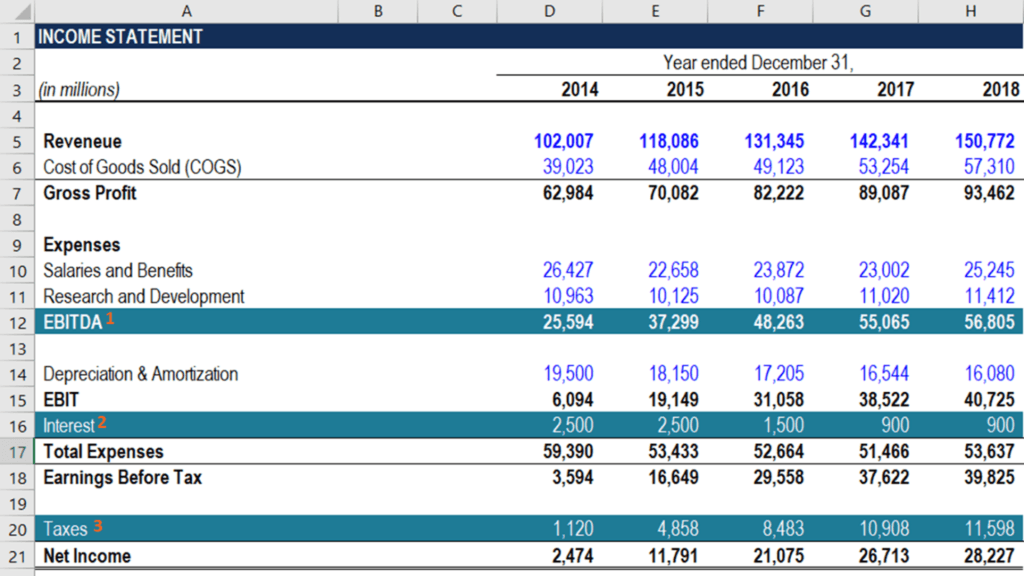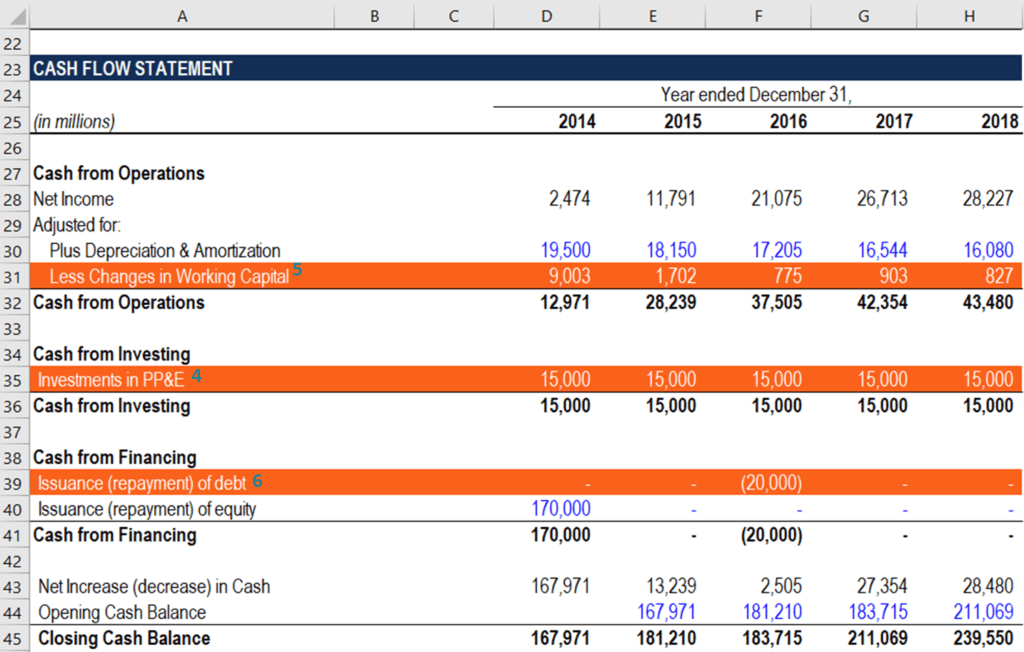# How to Calculate FCFE from EBITDA

FCFE = EBITDA – Interests – Taxes – ΔWorking Capital – CapEx + Net Borrowing

## How to Calculate FCFE from EBITDA?

You can calculate FCFE from EBITDA, by subtracting from it interest, taxes, change in net working capital, and capital expenditures – and then add net borrowing.

Free Cash Flow to Equity (FCFE) is the amount of cash generated by a company that can be potentially distributed to the company’s shareholders. FCFE is a crucial metric in one of the methods in the Discounted Cash Flow (DCF) valuation model. Using the FCFE, an analyst can determine the Net Present Value (NPV) of a company’s equity, which can be subsequently used to calculate the theoretical share price of the company.

The FCFE is different from the Free Cash Flow to Firm (FCFF), which indicates the amount of cash generated to all holders of the company’s securities (both investors and lenders). The formula below can be used to calculate FCFE from EBITDA:

#### FCFE = EBITDA – Interest – Taxes – ΔWorking Capital – CapEx + Net Borrowing

##### CapEx – Capital Expenditure### FCFE from EBITDA Formula

Earnings Before Interest, Taxes, Depreciation & Amortization (EBITDA) is one of the most commonly used metrics of a company’s profitability. Similar to Earnings Before Interest and Taxes (EBIT), EBITDA primarily assesses the company’s profitability from regular business activities. However, unlike EBIT, EBITDA also excludes depreciation and amortization expenses, providing a better overview of the operating profitability.

The EBITDA is one of the components for calculating a company’s net income. Therefore, one of the approaches to determining the free cash flow to equity includes the use of the EBITDA metric. Recall that the company’s net income is related to EBITDA through the following equation:

##### Net Income = EBITDA – Interest – Taxes – Depreciation & Amortization

Thus, we can substitute net income in the FCFE from the net income formula with the equation above:

##### Depreciation & Amortization – ΔWorking Capital – CapEx + Net Borrowing

In addition, the formula above can be simplified by removing the two depreciation and amortization variables with opposite signs:

##### FCFE = EBITDA – Interest – Taxes – ΔWorking Capital – CapEx + Net Borrowing

Where:

• FCFE – Free Cash Flow to Equity
• EBITDA – Earnings Before Interests, Taxes, Depreciation, and Amortization
• ΔWorking Capital – Change in the Working Capital
• CapEx – Capital Expenditure

The above approach of calculating free cash flow to equity provides a more detailed overview of the composition of the FCFE. Note that such a level of granularity is not always required in a financial model. In some cases, it can result in negative effects, as it complicates the comprehension of a model.

However, it is acceptable to apply this variation of the FCFE calculation when the assessment of the company’s profitability from its regular business activities (excluding other expenses) is essential.### FCFE from EBITDA Formula and Financial Statements

An analyst who calculates the free cash flows to equity in a financial model must be able to quickly navigate through the financial statements. The primary reason is that all the inputs required for the calculation of the metric are taken from the financial statements. The guidance below will help you to quickly and correctly incorporate the FCFE from EBITDA calculation into a financial model.

1. EBITDA: The company’s earnings before interests, taxes, depreciation, and amortization (EBITDA) are recorded on the company’s income statement.
2. Interest: The company’s interest expenses are located on the income statement after the EBIT.
3. Taxes: The tax payments can also be found on the income statement after the earnings before taxes (EBT).
4. CapEx: Capital expenditure (CapEx) can be found on the cash flow statement within the Cash from Investing section.
5. Change in Working Capital (can be also denoted as ΔWorking Capital) is calculated in the company’s cash flow statement within the Cash from Operations section.
6. Net Debt: The net debt amount is also located on the cash flow statement under the Cash from Investing section.

### More Resources

Thank you for reading CFI’s explanation of how to calculate FCFE from EBITDA. CFI is the official provider of the global Financial Modeling & Valuation Analyst (FMVA)™ certification program, designed to help anyone become a world-class financial analyst. To keep advancing your career, the additional CFI resources below will be useful:

• EBIT vs EBITDA Guide
• Projecting Income Statement Line Items
• Relative Valuation Models
• Statement of Cash Flows